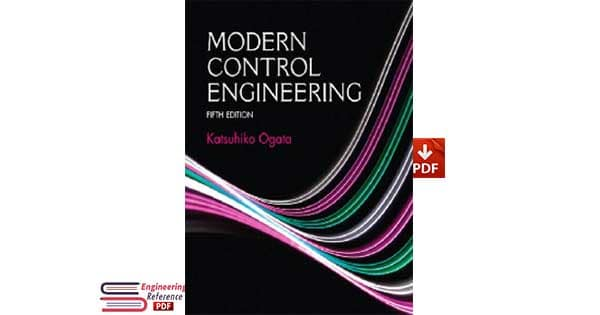# Modern Control Engineering Fifth Edition By Katsuhiko Ogata## preface of Modern Control Engineering Fifth Edition By Katsuhiko Ogata

This book introduces important concepts in the analysis and design of control systems. Readers will find it to be a clear and understandable textbook for control system courses at colleges and universities. It is written for senior electrical, mechanical, aerospace, or chemical engineering students. The reader is expected to have fulfilled the following prerequisites: introductory courses on differential equations, Laplace transforms, vector- matrix analysis, circuit analysis, mechanics, and introductory thermodynamics.

The main revisions made in this edition are as follows:

• The use of MATLAB for obtaining responses of control systems to various inputs has been increased. • The usefulness of the computational optimization approach with MATLAB has been demonstrated.

• New example problems have been added throughout the book.

• Materials in the previous edition that are of secondary importance have been deleted in order to provide space for more important subjects. Signal flow graphs were dropped from the book. A chapter on Laplace transform was deleted. Instead, Laplace transform tables, and partial-fraction expansion with MATLAB are pre- sented in Appendix A and Appendix B, respectively.

• A short summary of vector-matrix analysis is presented in Appendix C; this will help the reader to find the inverses of n x n matrices that may be involved in the analy- sis and design of control systems.